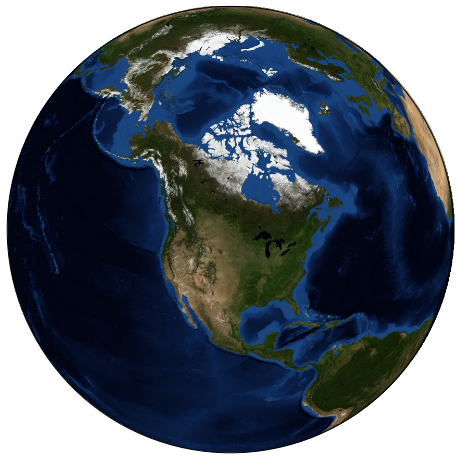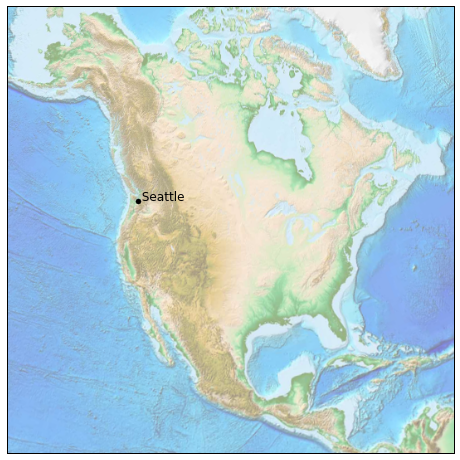This notebook contains an excerpt from the Python Data Science Handbook by Jake VanderPlas; the content is available on GitHub.

The text is released under the CC-BY-NC-ND license, and code is released under the MIT license. If you find this content useful, please consider supporting the work by buying the book!

# Geographic Data with Basemap¶

One common type of visualization in data science is that of geographic data. Matplotlib's main tool for this type of visualization is the Basemap toolkit, which is one of several Matplotlib toolkits which lives under the mpl_toolkits namespace. Admittedly, Basemap feels a bit clunky to use, and often even simple visualizations take much longer to render than you might hope. More modern solutions such as leaflet or the Google Maps API may be a better choice for more intensive map visualizations. Still, Basemap is a useful tool for Python users to have in their virtual toolbelts. In this section, we'll show several examples of the type of map visualization that is possible with this toolkit.

Installation of Basemap is straightforward; if you're using conda you can type this and the package will be downloaded:

\$ conda install basemap

We add just a single new import to our standard boilerplate:

In :
%matplotlib inline
import numpy as np
import matplotlib.pyplot as plt
from mpl_toolkits.basemap import Basemap


Once you have the Basemap toolkit installed and imported, geographic plots are just a few lines away (the graphics in the following also requires the PIL package in Python 2, or the pillow package in Python 3):

In :
plt.figure(figsize=(8, 8))
m = Basemap(projection='ortho', resolution=None, lat_0=50, lon_0=-100)
m.bluemarble(scale=0.5);The meaning of the arguments to Basemap will be discussed momentarily.

The useful thing is that the globe shown here is not a mere image; it is a fully-functioning Matplotlib axes that understands spherical coordinates and which allows us to easily overplot data on the map! For example, we can use a different map projection, zoom-in to North America and plot the location of Seattle. We'll use an etopo image (which shows topographical features both on land and under the ocean) as the map background:

In :
fig = plt.figure(figsize=(8, 8))
m = Basemap(projection='lcc', resolution=None,
width=8E6, height=8E6,
lat_0=45, lon_0=-100,)
m.etopo(scale=0.5, alpha=0.5)

# Map (long, lat) to (x, y) for plotting
x, y = m(-122.3, 47.6)
plt.plot(x, y, 'ok', markersize=5)
plt.text(x, y, ' Seattle', fontsize=12);This gives you a brief glimpse into the sort of geographic visualizations that are possible with just a few lines of Python. We'll now discuss the features of Basemap in more depth, and provide several examples of visualizing map data. Using these brief examples as building blocks, you should be able to create nearly any map visualization that you desire.

## Map Projections¶

The first thing to decide when using maps is what projection to use. You're probably familiar with the fact that it is impossible to project a spherical map, such as that of the Earth, onto a flat surface without somehow distorting it or breaking its continuity. These projections have been developed over the course of human history, and there are a lot of choices! Depending on the intended use of the map projection, there are certain map features (e.g., direction, area, distance, shape, or other considerations) that are useful to maintain.

The Basemap package implements several dozen such projections, all referenced by a short format code. Here we'll briefly demonstrate some of the more common ones.

We'll start by defining a convenience routine to draw our world map along with the longitude and latitude lines:

In :
from itertools import chain

def draw_map(m, scale=0.2):
# draw a shaded-relief image

# lats and longs are returned as a dictionary
lats = m.drawparallels(np.linspace(-90, 90, 13))
lons = m.drawmeridians(np.linspace(-180, 180, 13))

# keys contain the plt.Line2D instances
lat_lines = chain(*(tup for tup in lats.items()))
lon_lines = chain(*(tup for tup in lons.items()))
all_lines = chain(lat_lines, lon_lines)

# cycle through these lines and set the desired style
for line in all_lines:
line.set(linestyle='-', alpha=0.3, color='w')


### Cylindrical projections¶

The simplest of map projections are cylindrical projections, in which lines of constant latitude and longitude are mapped to horizontal and vertical lines, respectively. This type of mapping represents equatorial regions quite well, but results in extreme distortions near the poles. The spacing of latitude lines varies between different cylindrical projections, leading to different conservation properties, and different distortion near the poles. In the following figure we show an example of the equidistant cylindrical projection, which chooses a latitude scaling that preserves distances along meridians. Other cylindrical projections are the Mercator (projection='merc') and the cylindrical equal area (projection='cea') projections.

In :
fig = plt.figure(figsize=(8, 6), edgecolor='w')
m = Basemap(projection='cyl', resolution=None,
llcrnrlat=-90, urcrnrlat=90,
llcrnrlon=-180, urcrnrlon=180, )
draw_map(m)